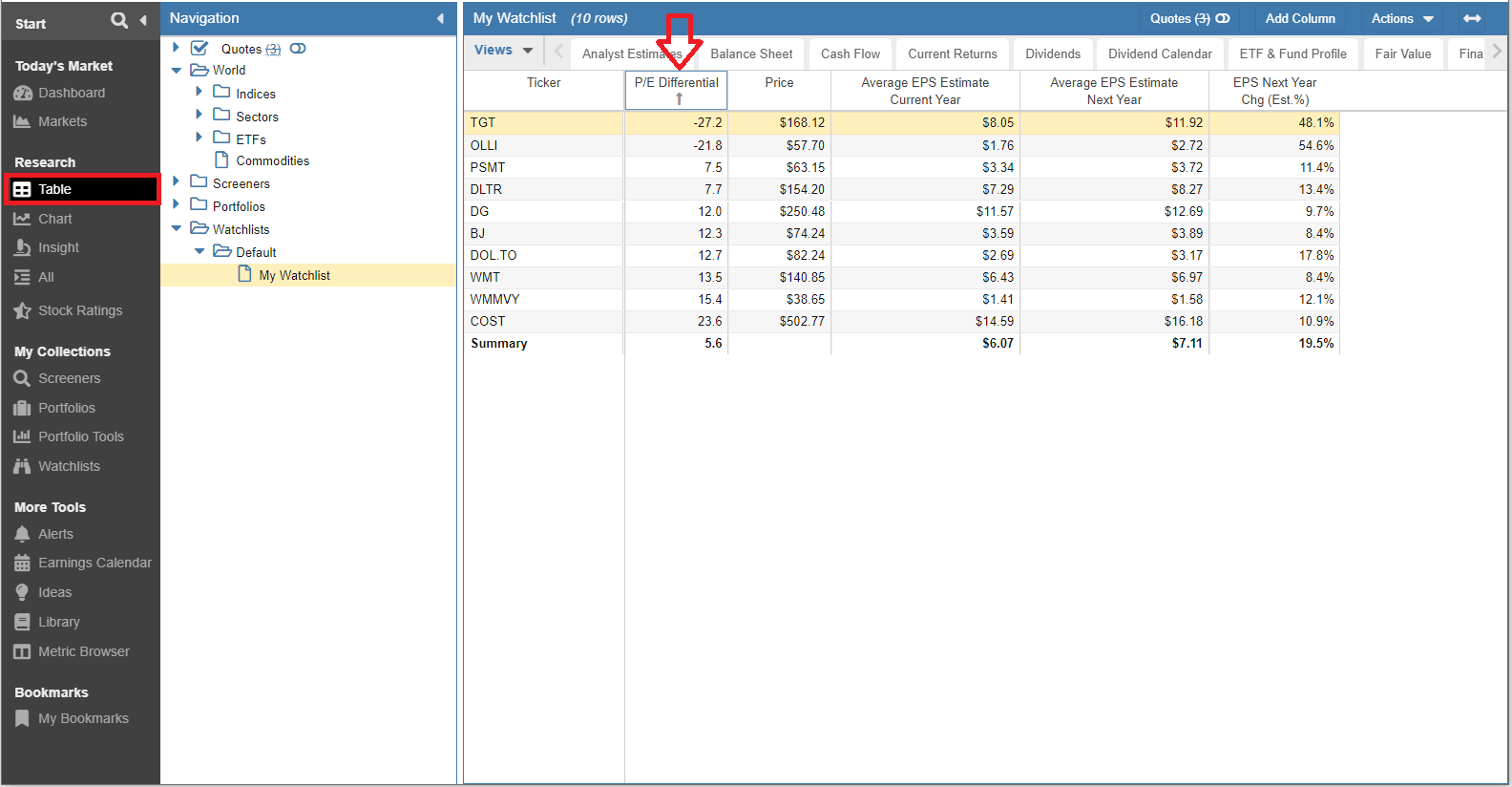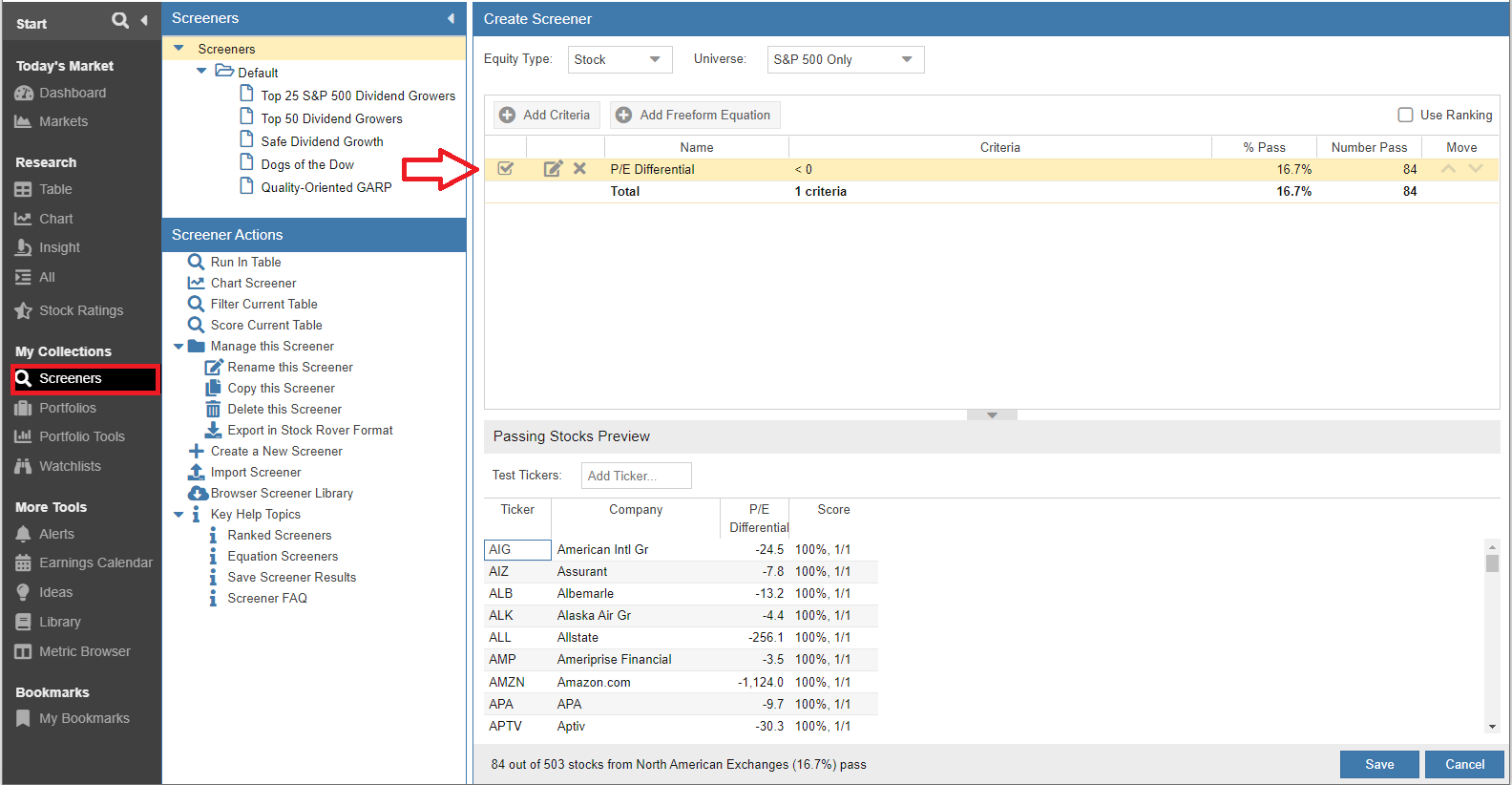# Quick and Dirty Way to Test if a Stock is Undervalued

September 15, 2022

When looking at any given stock, you might be thinking, “Is this stock overvalued or undervalued?”

That’s really the fundamental question, isn’t it?

One easy way to think about this is to look for stocks with long-term earnings growth forecasts that trade at a discount. Conversely, we should avoid stocks with low expectations for earnings growth that trade at a premium.

So, how do you then find companies that are trading at a discount to the market that have long-term earnings growth expectations?

I want to introduce you to the P/E Differential, which is the difference between a stock’s P/E ratio (a non-standard version of P/E that I will explain) and its expected earnings growth rate. The P/E Differential can tell you in broad strokes if a company is undervalued or overvalued relative to its expected future earnings.

The P/E Differential is available in the Metric Browser and can be found in the Valuation folder. Using the add column feature you can add the metric to a View the Table.

Let’s learn a bit more about how this metric is calculated.

### Components of the Calculation

You need two pieces of information to calculate the P/E Differential. Both are very simple to get.

1. Price-to-Earnings Ratio

We’re going to use a non-standard P/E for this. The problem with the way P/E ratios are expressed on most financial platforms is that they are presented as “rear view” numbers. That is, the P/E is based on what the company earned in the PREVIOUS 12 months—not what the company is expected to earn in the NEXT (future) 12 months. So we’re going to use a modified version of the standard ratio and take Price divided by the projected EPS for the current year—in Stock Rover this is called Average EPS Estimate Current Year.

Since you are an investor, you are not looking to buy “today’s” valuation. You are looking to buy the future value of a company. This brings us to the second component to our P/E Differential calculation…

2. Earnings Growth

The earnings growth rate tells you how quickly a company is expected to grow its earnings or profits. Earnings estimates, provided by market analysts, forecast out four quarters of earnings and two years of earnings. From those numbers, we can project the rate at which a company is expected to grow those earnings.

When we put the two numbers together we can get a forward-looking P/E ratio that can tell us whether a company is overvalued or undervalued.

### P/E Differential Calculation

Forward Looking P/E Ratio – Earnings Growth Rate = P/E Differential

• A negative number means that the company is undervalued relative to the future earnings growth which signals a buying opportunity or further analysis.
• A positive number means that the company is overvalued relative to the future earnings growth which tells us to not buy the stock, or to sell the stock if we hold a position.

To calculate the P/E differential number, we will need the following:

• Current Price
• Average EPS Estimate Current Year
• Average EPS Estimate Next Year

For Example, let’s use Target (TGT) as an example.

What You Need Price
Current Price \$168.12
Average EPS Estimate Current Year \$8.05
Average EPS Estimate Next Year \$11.92

Let’s calculate the P/E Differential:

1. First, calculate the P/E Ratio (Current Price / Average EPS Estimate Current Year)

`\$168.12 / \$8.05 = 20.88 `

2. Now we need to calculate the Earnings Growth Rate:

To get this number we will need to divide the Average EPS Estimate Next Year by the Average EPS Estimate Current Year:

`\$11.92 / \$8.05 = 1.481`

3. Next subtract 1 to get the percentage growth rate:

`1.481 - 1 = 48.1%`

4. Finally, we subtract the growth rate from the P/E:

` 20.88 (P/E ratio) – 48.1 (Earnings Growth Rate) = - 27.2`

When a negative number is returned, this indicates that the stock is undervalued!

NOTE: This is just one data point. I always encourage additional evaluation to ensure that your stock is fundamentally a strong company. You can do this by reading the quarterly and annual reports and doing further analysis with Stock Rover.

Keep in mind, if we buy solid, high-quality, and well-managed companies at valuations that we deem to be a good buy, then it makes no difference where the general market goes this year or next. We simply do not worry about it. Continually applying this strategy, buying great companies on sale, we can’t help but make great returns over the long term.

### Using P/E Differential in Stock Rover

You can easily add the P/E Differential metric to a View in the Table.

Below is a sampling of the P/E Differentials for Target and some of its peers. For reference, the Table also includes the columns used in the P/E Differential calculation:

I’ve sorted on P/E Differential, which makes it easy to see what’s the most negative (undervalued).You can also screen on the P/E Differential! Just search for companies with a P/E Differential less than 0 and you’ll only find undervalued companies (according to this one metric).### Conclusion

The P/E Differential valuation method is a good, quick method to test whether a stock’s current price is reasonable or not. While in most cases it is imprecise, honestly ANY stock valuation method is by nature imprecise. With just a few pieces of information, you can determine if a stock is undervalued or overvalued relative to its future earnings. Add the P/E Differential method to your toolbox for finding attractive stock opportunities.